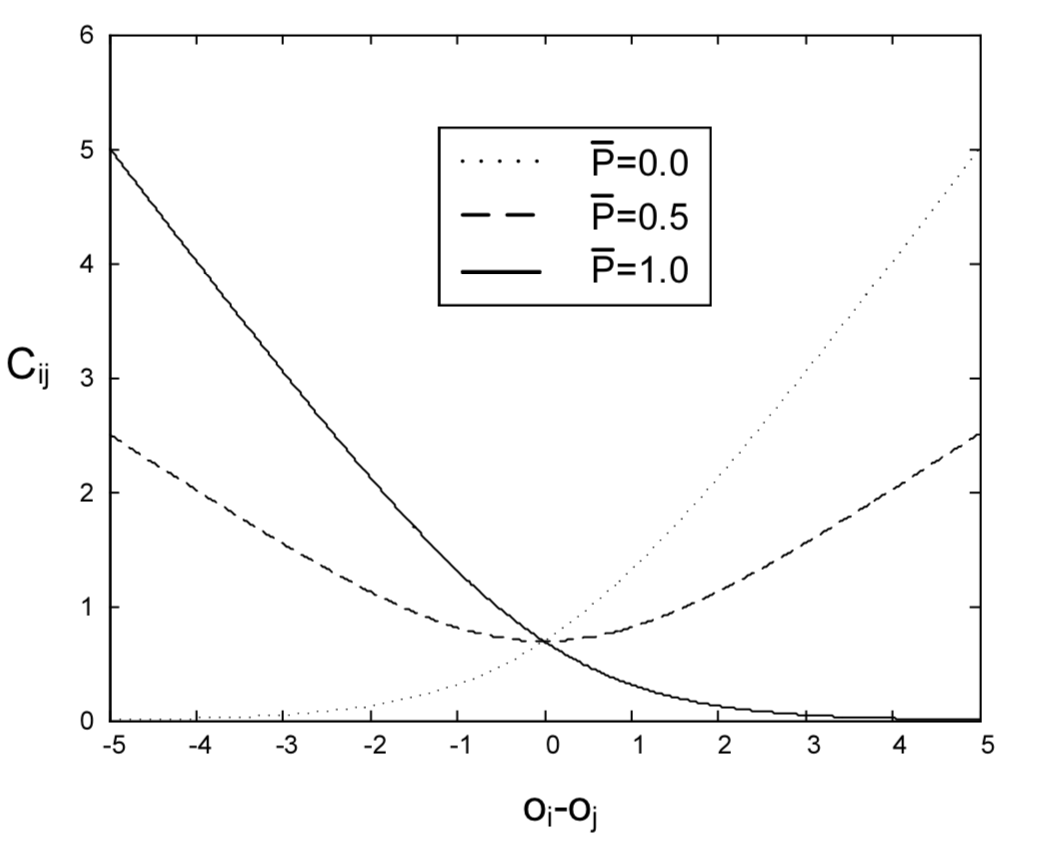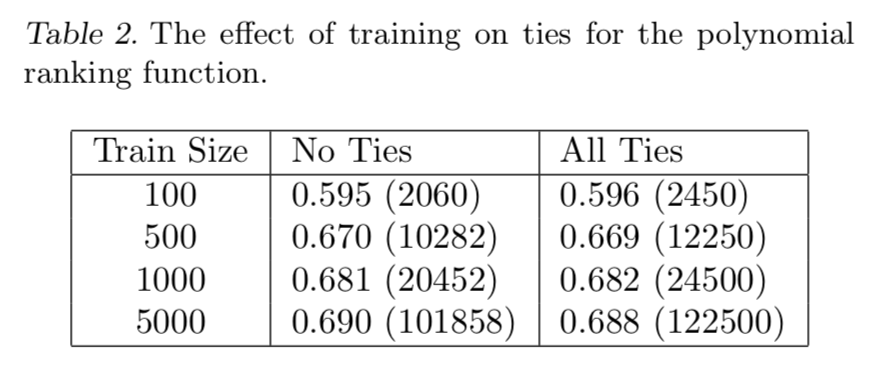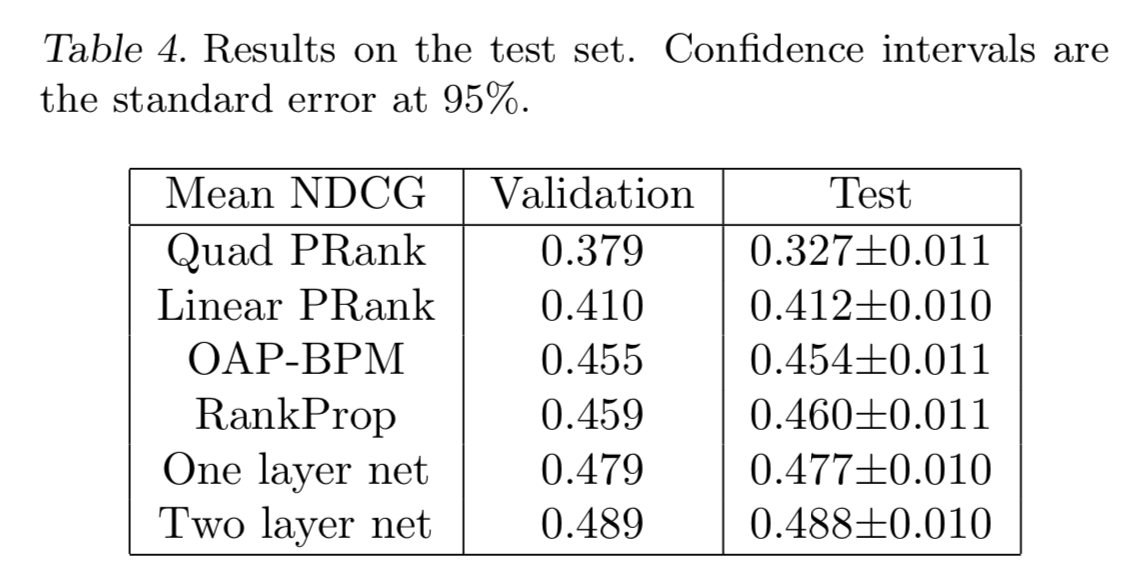0%

## Introduction

cast the ranking problem as an ordinal regression problem is to solve an unnecessarily hard problem, we don’t need to do this

we call the resulting algorithm, together with the probabilistic cost function we describe below —— RankNet

## Previous Work

### RankProp

(Caruana et al., 1996)

• adv:
• point-wise —— O(m) time complexity
• dis:
• it is not known under what conditions it converges
• it does not give a probabilistic model

### PRank

(Herbrich et al., 2000)

• adv:
• point-wise —— O(m) time complexity
• dis:
• outperform by another version

### “general framework”

(Dekel et al., 2004)

• dis:
• no probabilistic model

### RankBoost

(Freund et al., 2003)

similar with this paper but use decision stumps as the weak learners

## A Probabilistic Ranking Cost Function

We consider models $f : R^d → R$ such that the rank order of a set of test samples is specified by the real values that f takes

Denote the modeled posterior $P(x_i > x_j)$ by $P_{ij}$:

which $o_{ij} = f(x_i) - f(x_j)$

and use cross entropy cost function:

for problems with noisy labels this is likely to be more robust than a quadratic cost### Combining Probabilities

If we have $\bar P_{ij}, \bar P_{jk}$ then we have:

## RankNet: Learning to Rank with Neural Nets

Prove RankNet with Neural Nets is different differentiable

skip prove detail

## Experiments on Artificial DataWhether there are ties in training data, results are very close

## Experiments on Real Data

Ranking accuracy was computed using a normalized discounted cumulative gain measure（NDCG）:

where $N_i$ is a normalization constant so that a perfect ordering gets NDCG score 1
$r(j)$ is the rating of the j’th document## Discussion

Can these ideas be extended to the kernel learning framework?

depending on the kernel, the objective function may not be convex

skip